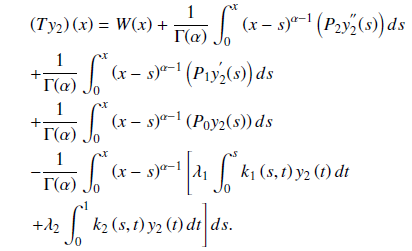# Collocation Approach for the Computational Solution Of Fredholm-Volterra Fractional Order of Integro-Differential Equations

## Authors

• Ganiyu Ajileye Department of Mathematics and Statistics, Federal University Wukari, Taraba State, Nigeria.
• Adewale James Department of Mathematics, American University of Nigeria, Yola, Adamawa State, Nigeria.
• Ayinde Abdullahi Department of Mathematics, Moddibo-Adama University, Yola Nigeria.
• Taiye Oyedepo Federal College of Dental Technology and Therapy, Enugu, Nigeria.

## Keywords:

FREDHOLM-VOLTERRA, Collocation, Integro-differential

## Abstract

In this work, a collocation technique is used to determine the computational solution to fractional order Fredholm-Volterra integro-differential equations with boundary conditions using Caputo sense. We obtained the linear integral form of the problem and transformed it into a system of linear algebraic equations using standard collocation points. The matrix inversion approach is adopted to solve the algebraic equation and substituted it into the approximate solution. We established the uniqueness and convergence of the method and some modelled numerical examples are provided to demonstrate the method’s correctness and efficiency. It is observed that the results obtained by our new method are accurate and performed better than the results obtained in the literature. The study will be useful to engineers and scientists. It is advantageous because it addresses the difficulty in tackling fractional order Fredholm-Volterra integro-differential problems using a simple collocation strategy. The approach has the advantage of being more accurate and reducing computer running time.

Dimensions

P. Kamal, Higher order numerical methods for fractional order differential equations, Doctoral dissertation, University of Chester, United Kingdom, 2015. http://hdl.handle.net/10034/613354.

D. Baleanu, K. Diethelm & J. J. Trujillo, Fractional calculus: Models and numerical methods, Series on complexity nonlinearity and chaos, World Scientific 2012. DOI: https://doi.org/10.1142/8180

R. H. Khan & H. O. Bakodah, “Adomian decomposition method and its modification for nonlinear Abel’s integral equations”, Computers and Mathematics with Applications 7 (2013) 2349. DOI: https://doi.org/10.12988/ijma.2013.37179

R. C. Mittal & R. Nigam, “Solution of fractional integro-differential equations by Adomiandecomposition method”, The International Journal of Applied Mathematics and Mechanics 2 (2008) 87.

S. Momani & M. A. Noor, “Numerical methods for fourth-order fractional integro-differential equations”, Applied Mathematics and Computation 1 (2006) 754. DOI: https://doi.org/10.1016/j.amc.2006.04.041

A. O. Agbolade & T. A. Anake, “Solution of first order volterra linear integro differential equations by collocation method”, J. Appl. Math., Article ID. 1510267 (2017). doi:10.1155/2017/1510267 DOI: https://doi.org/10.1155/2017/1510267

O. Cem, S. Mehmet & D. O. Arzu, “Chelyshkov collocation approach to solving the systems of linear functional differential equations”, New Trend in Mathematical Sciences (NTMSCI) 4 (2015) 83.

D. Nazari & M. Jahanshahi, “Some notes on multi-order frational integro-differential equations”, 43rd Annual Iranian Mathematics Conference (2012) pp 27.

D. S. Mohammed, ”Numerical solution of fractional integro-differential equations by least square method and shifted Chebyshev polynomial”, Mathematical Problems in Engineering 2014 (2014) 431965. https://doi.org/10.1155/2014/431965 DOI: https://doi.org/10.1155/2014/431965

L. Huang, X. F. Li, Y. Zhao & X. Y. Duan, “Approximate solution of fractional integro-differential equations by Taylor expansion method”, Computers & Mathematics with Applications 3 (2011) 1127. DOI: https://doi.org/10.1016/j.camwa.2011.03.037

A. Arikoglu & I. Ozkol, ”Solution of fractional integro-differential equations by using fractional differential transform method”, Chaos, Solitons & Fractals 2 (2009) 521. DOI: https://doi.org/10.1016/j.chaos.2007.08.001

A. A. Elbeleze, A. Klman & B. M. Taib, “Approximate solution of integro-differential equation of fractional (arbitrary) order”, Journal of King Saud University - Science 28 (2016) 61. DOI: https://doi.org/10.1016/j.jksus.2015.04.006

Y. Nawaz, “Variational iteration method and homotopy perturbation method for fourth-order fractional integro-differential equations”, Computers & Mathematics with Applications 8 (2011) 2330. DOI: https://doi.org/10.1016/j.camwa.2010.10.004

R. K. Saeed & H. M. Sdeq, “Solving a system of linear fredholm fractional integro-differential equations using homotopy perturbation method”, Australian Journal of Basic and Applied Sciences 4 (2010) 633.

S. Alkan, “A new solution method for nonlinear fractional integro-differential equations”, Discrete and Continuous Dynamical Systems - Series S 6 (2015) 1065. DOI: https://doi.org/10.3934/dcdss.2015.8.1065

S. Alkan & A. Secer, “Solution of nonlinear fractional boundary value problems with nonho-mogeneous boundary conditions”, Applied and Computational Mathematics 3 (2015).

S. Alkan & V. F. Hatipoglu, “Approximate solutions of Volterra-Fredholm integro-differential equations of fractional order”, Tbilisi Mathematical Journal 2 (2017) 1. DOI: https://doi.org/10.1515/tmj-2017-0021

O. A. Uwaheren, A. F. Adebisi & O. A. Taiwo, “Perturbed Collocation Method For Solving Singular Multi-order Fractional Differential Equations of Lane-Emden Type”, Journal of the Nigerian Society of Physical Sciences 3 (2020) 141. https://doi.org/10.46481/jnsps.2020.69. DOI: https://doi.org/10.46481/jnsps.2020.69

I. Avc & N. I. Mahmudov, “Numerical Solutions for Multi-Term 7 Fractional Order Differential Equations with Fractional Taylor Operational Matrix of Fractional Integration”, Mathematics 1 (2020) 96. https://doi.org/10.3390/math8010096. DOI: https://doi.org/10.3390/math8010096

L. Linna, W. Zhirou & H. Qiongdan, “A numerical method for solving feactional variational problems by operational matrix based on Chelyshekov polynomial”, Enginerring Letters 28 (2020) 486.

S. E. Fadugba, “Solution of Fractional Order Equations in the Domain of the Mellin Transform”, Journal of the Nigerian Society of Physical Sciences 4 (2019) 138. https://doi.org/10.46481/jnsps.2019.31 DOI: https://doi.org/10.46481/jnsps.2019.312022-10-03

## How to Cite

Ajileye, G. ., James, A., Abdullahi, A. ., & Oyedepo, T. (2022). Collocation Approach for the Computational Solution Of Fredholm-Volterra Fractional Order of Integro-Differential Equations. Journal of the Nigerian Society of Physical Sciences, 4(4), 834. https://doi.org/10.46481/jnsps.2022.834

## Section

Original Research